# OCR：CRNN+CTC開源加詳細解析

Posted on October 12, 2020 in AI.ML. View: 8,334

## 開源：pytorch版本的CRNN+CTC

GitYCC/crnn-pytorch

👆🏼👆🏼👆🏼👆🏼👆🏼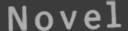## 網路架構

CRNN+CTC 結構源於論文An End-to-End Trainable Neural Network for Image-based Sequence Recognition and Its Application to Scene Text Recognition (2015), Baoguang Shi et al.，其網路架構其實並不複雜，講白了就是 CNN 的 Backbone 再搭配 Bi-directional RNN，最後對每個時間點作Softmax分類問題，但是在衡量輸出時則是需要綜觀每個時間點的 CTC Loss。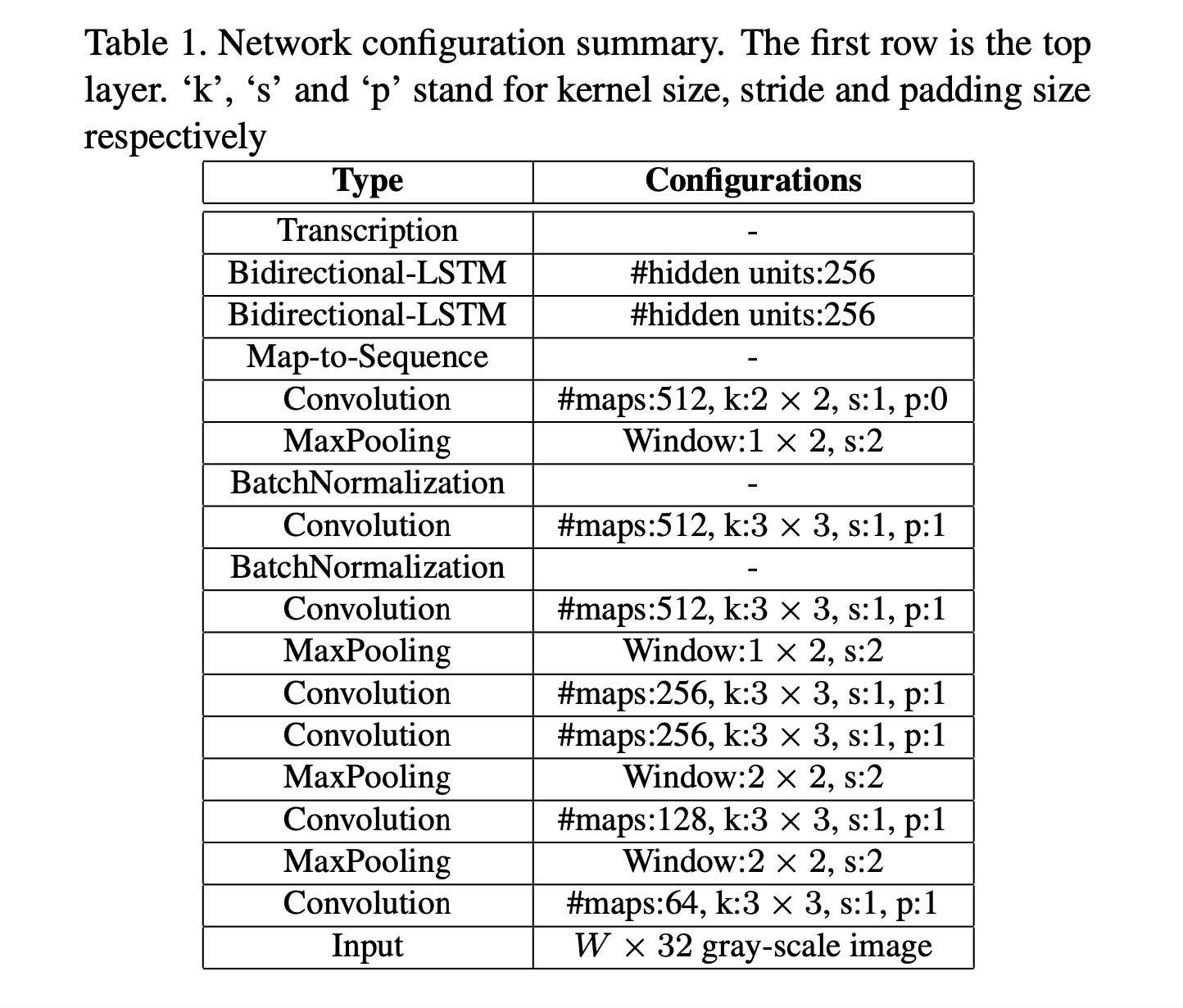1 2 3 4 5 6 7 8 9 10 11 12 13 14 15 16 17 18 19 20 21 22 23 24 25 26 27 28 29 30 31 32 33 34 35 36 37 38 39 40 41 42 43 44 45 46 47 48 49 50 assert img_height % 16 == 0 assert img_width % 4 == 0 channels = [img_channel, 64, 128, 256, 256, 512, 512, 512] kernel_sizes = [3, 3, 3, 3, 3, 3, 2] strides = [1, 1, 1, 1, 1, 1, 1] paddings = [1, 1, 1, 1, 1, 1, 0] cnn = nn.Sequential() def conv_relu(i, batch_norm=False): # shape of input: (batch, input_channel, height, width) input_channel = channels[i] output_channel = channels[i+1] cnn.add_module( f'conv{i}', nn.Conv2d(input_channel, output_channel, kernel_sizes[i], strides[i], paddings[i]) ) if batch_norm: cnn.add_module(f'batchnorm{i}', nn.BatchNorm2d(output_channel)) relu = nn.LeakyReLU(0.2, inplace=True) if leaky_relu else nn.ReLU(inplace=True) cnn.add_module(f'relu{i}', relu) # size of image: (channel, height, width) = (img_channel, img_height, img_width) conv_relu(0) cnn.add_module('pooling0', nn.MaxPool2d(kernel_size=2, stride=2)) # (64, img_height // 2, img_width // 2) conv_relu(1) cnn.add_module('pooling1', nn.MaxPool2d(kernel_size=2, stride=2)) # (128, img_height // 4, img_width // 4) conv_relu(2) conv_relu(3) cnn.add_module( 'pooling2', nn.MaxPool2d(kernel_size=(2, 1)) ) # (256, img_height // 8, img_width // 4) conv_relu(4, batch_norm=True) conv_relu(5, batch_norm=True) cnn.add_module( 'pooling3', nn.MaxPool2d(kernel_size=(2, 1)) ) # (512, img_height // 16, img_width // 4) conv_relu(6) # (512, img_height // 16 - 1, img_width // 4 - 1) 

 1 self.map_to_seq = nn.Linear(output_channel * output_height, map_to_seq_hidden) 

 1 2 3 conv = conv.view(batch, channel * height, width) conv = conv.permute(2, 0, 1) # (width, batch, feature) seq = self.map_to_seq(conv)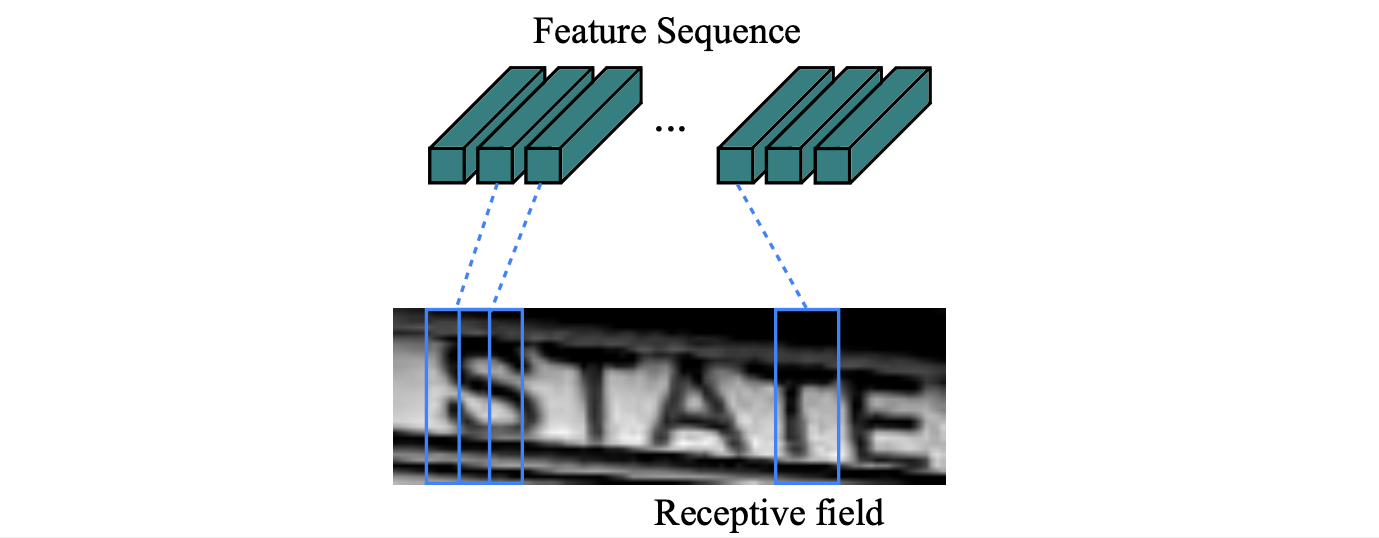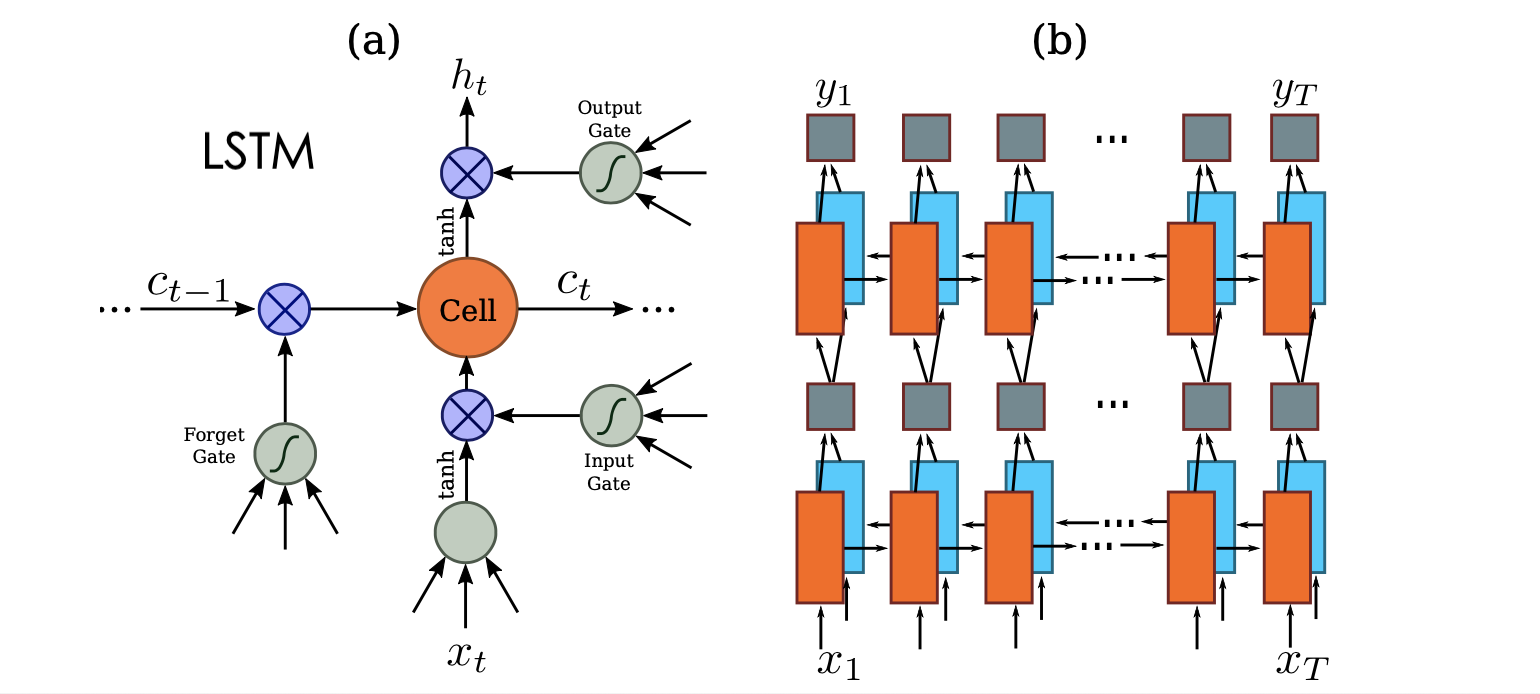## CTC (Connectionist Temporal Classification)

RNNs 與其他傳統方法相比，如：HMMs (Hidden Markov Model) 和 CRFs (Conditional Random Field) 有以下優勢：

• RNNs 無須多餘的先驗假設
• RNNs 提供了相當強大且一般化的機制去描述時間序列

CTC 設計了一個機制讓我們可以做到這件事：

1. 在原本要預測的字元中多加入了 blank ε
2. 透過映射機制 $$B$$ 將 RNN 的輸出轉化成 Sequence Prediction
3. 映射機制 $$B$$：合併相鄰的字元並且除去 blank ε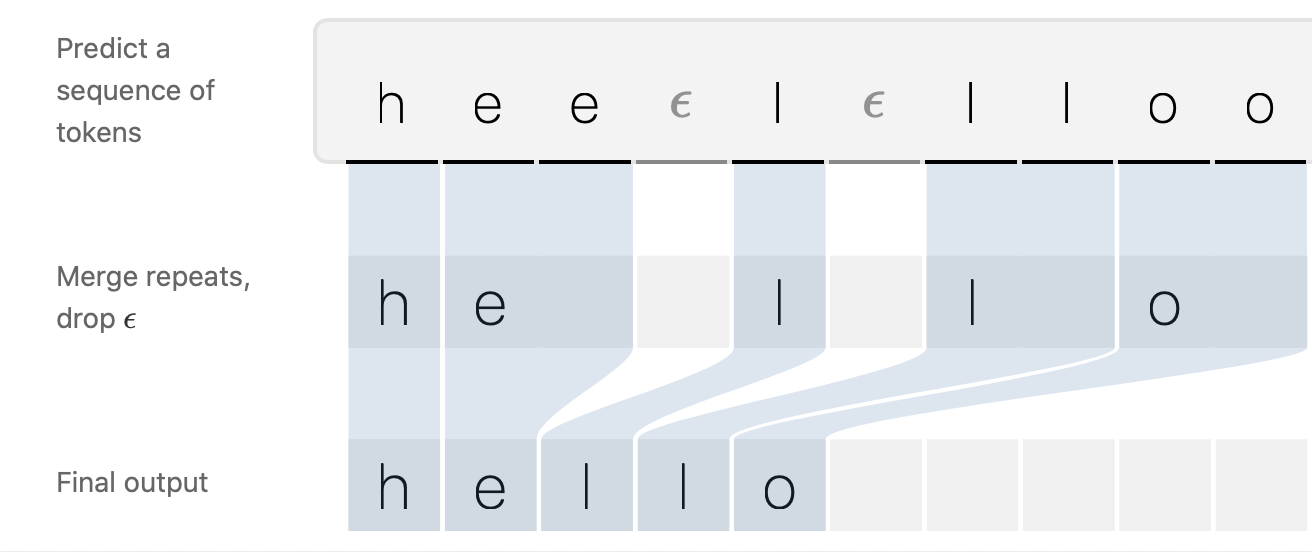## CTC Loss: Forward-Backward Algorithm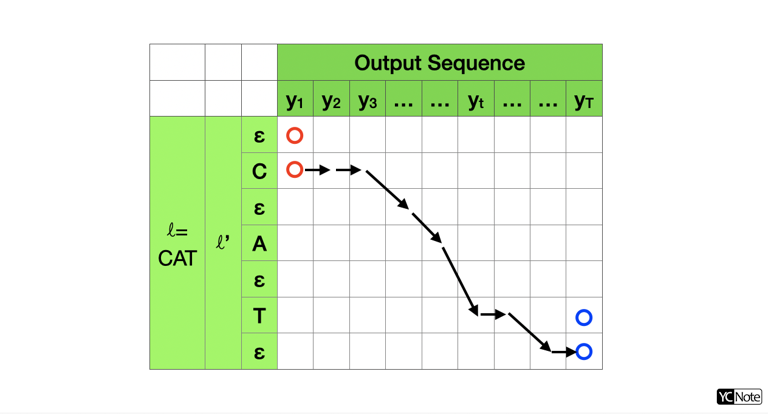$$p(\ell|y)=\sum_{π:B(π)=\ell}p(π|y)\ \text{ ↪︎【1】}$$

$$p(π|y)=\prod_{t=1}^{T}y^{t}_{π_t}\ \text{ ↪︎【2】}$$

$$y^{t}_{π_t}$$ 表示在時間 $$t$$ Label 為 $$π_t$$ 的機率。

$$min_{y}\ -ln[p(\ell|y)]\ \text{ ↪︎【3】}$$

$$\frac{\partial}{\partial y}\{ -ln[p(\ell|y)]\}=\frac{-1}{p(\ell|y)}\frac{\partial p(\ell|y)}{\partial y}\ \text{ ↪︎【4】}$$

$$\alpha_t(s)\equiv\sum_{π:B(π_{1:t})=\ell_{1:s}}\prod_{t'=1}^{t}y^{t'}_{π_{t'}}\ \text{ ↪︎【5】}$$

$$\alpha_t(s)$$ 表示從可能的起始點累積到 $$t$$ 時刻且 Label $$\ell'=s$$ 那點的總機率，可以從式子的右式看出，加總所有能將 $$π_{1:t}$$ (圖一中的衡欄) 映射到 $$\ell_{1:s}$$ (圖一中的縱欄) 的路徑 $$\pi$$ ，並且將這路徑 $$\pi$$ 上的各點機率相乘 (因為互為獨立事件)。

$$p(\ell|y)=\alpha_T(|\ell'|)+\alpha_T(|\ell'|-1)\ \text{ ↪︎【6】}$$

$$\begin{cases} \alpha_1(1)=y_\epsilon^1 \\ \alpha_1(2)=y_{\ell_1}^1 \\ \alpha_1(s)=0,\ \forall s> 2 \end{cases}\ \text{ ↪︎【7】}$$

$$\alpha_t(s)=[\sum_{π:B(π_{1:t-1})=\ell_{1:s}}\prod_{t'=1}^{t}y^{t'}_{π_{t'}}]y^{t}_{l'_{s}}=[\sum_{s'\in connected\ to\ (t,s)}\alpha_{t-1}(s')]y^{t}_{l'_{s}}\ \text{ ↪︎【8】}$$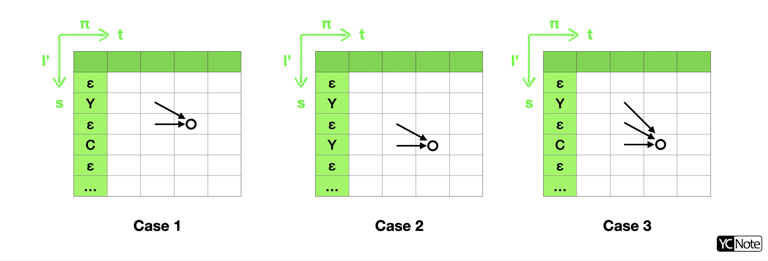$$\alpha_t(s)= \begin{cases} [\alpha_{t-1}(s)+\alpha_{t-1}(s-1)]y_{l'_s}^t& \text{if } l'_s=\epsilon\text{ or }l'_{s-2}=l'_s\\ [\alpha_{t-1}(s)+\alpha_{t-1}(s-1)+\alpha_{t-1}(s-2)]y_{l'_s}^t & \text{otherwise} \end{cases}\ \text{ ↪︎【9】}$$

$$\beta_t(s)\equiv\sum_{π:B(π_{t:T})=\ell_{s:|\ell|}}\prod_{t'=t}^{T}y^{t'}_{π_{t'}}\ \text{ ↪︎【10】}$$

$$\begin{cases} \beta_T(|\ell'|)=y_\epsilon^T \\ \beta_T(|\ell'|-1)=y_{\ell_{|\ell|}}^T \\ \beta_T(s)=0,\ \forall s< |\ell'|-1 \end{cases}\ \text{ ↪︎【11】}$$

$$\beta_t(s)=y^{t}_{l'_{s}}[\sum_{π:B(π_{t:T})=\ell_{s:|\ell|}}\prod_{t'=t+1}^{T}y^{t'}_{π_{t'}}]=y^{t}_{l'_{s}}[\sum_{s'\in connected\ from\ (t,s)}\beta_{t+1}(s')]\ \text{ ↪︎【12】}$$

$$(t,s)$$ 連接的情況一樣也可分為三種情況，如下圖：$$\beta_t(s)= \begin{cases} [\beta_{t+1}(s)+\beta_{t+1}(s+1)]y_{l'_s}^t& \text{if } l'_s=\epsilon\text{ or }l'_{s+2}=l'_s\\ [\beta_{t+1}(s)+\beta_{t+1}(s+1)+\beta_{t+1}(s+2)]y_{l'_s}^t & \text{otherwise} \end{cases}\ \text{ ↪︎【13】}$$

$$\alpha_t(s)\beta_t(s)=\sum_{π:B(π)=\ell\ \&\ \pi_t=\ell'_s}\ y_{\ell'_s}^t\prod_{t'=1}^{T}y^{t'}_{π_{t'}}\ \text{ ↪︎【14】}$$

$$\Rightarrow \frac{\alpha_t(s)\beta_t(s)}{y_{\ell'_s}^t}=\sum_{π:B(π)=\ell\ \&\ \pi_t=\ell'_s}\ \prod_{t'=1}^{T}y^{t'}_{π_{t'}}＝\sum_{π:B(π)=\ell\ \&\ \pi_t=\ell'_s}p(π|y)\ \text{ ↪︎【15】}$$

$$p(\ell|y)=\sum_{s=1}^{|\ell'|}\sum_{π:B(π)=\ell\ \&\ \pi_t=\ell'_s}p(π|y)=\sum_{s=1}^{|\ell'|}\frac{\alpha_t(s)\beta_t(s)}{y_{\ell'_s}^t}\ \text{ ↪︎【16】}$$

【16】式提供了另外一個求 $$p(\ell|y)$$ 的方法，而且這個算法可以在任意時間點 $$t$$ 作計算，更棒的是它可以讓我們很方便的求得 $$\frac{\partial p(\ell|y)}{\partial y}$$

$$\frac{\partial p(\ell|x)}{\partial y_k^t}=\frac{\partial}{\partial y_k^t}[\sum_{s=1}^{|\ell'|}\frac{\alpha_t(s)\beta_t(s)}{y_{\ell'_s}^t}]\ \text{ ↪︎【17】}$$

$$=\frac{\partial}{\partial y_k^t}[\sum_{s\in \{\ell'_s=k\}}\frac{\alpha_t(s)\beta_t(s)}{y_{\ell'_s}^t}]$$
$$=\frac{\partial}{\partial y_k^t}[\sum_{s\in \{\ell'_s=k\}}\frac{(\cdots)y_{l'_s}^t\times y_{l'_s}^t(\cdots)}{y_{l'_s}^t}]$$
$$=\sum_{s\in \{\ell'_s=k\}}\frac{(\cdots)y_{l'_s}^t\times y_{l'_s}^t(\cdots)}{[y_{l'_s}^{t}]^2}$$
$$\Rightarrow \frac{\partial p(\ell|x)}{\partial y_k^t}=\frac{1}{[y_{l'_s}^{t}]^2}\sum_{s\in \{\ell'_s=k\}}\alpha_t(s)\beta_t(s)\ \text{ ↪︎【18】}$$

## Inference of CTC

GitYCC/crnn-pytorch 中我有實作了三種 CTC 的 Inference 方法，分別為 greedy_decodebeam_search_decodeprefix_beam_decode，精確度依序越來越準確，但是隨著精確度提高所花的 Inference 時間也就越長。

### Greedy Decode

$$\ell^*\approx B(\pi^*)\ ;\ \pi^*= \{argmax_{s}\ y^{t}_{s}\ \text{ for t=1..T}\}$$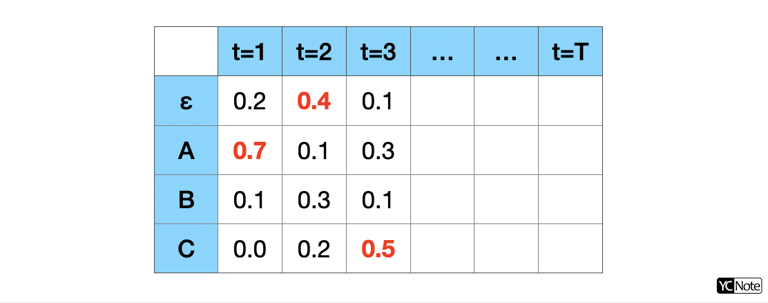1 2 3 4 def greedy_decode(emission_log_prob, blank=0, **kwargs): labels = np.argmax(emission_log_prob, axis=-1) labels = _reconstruct(labels, blank=blank) return labels 

### Beam Search Decode1 2 3 4 5 6 7 8 9 10 11 12 13 14 15 16 17 18 19 20 21 22 23 24 25 26 27 28 29 30 31 32 33 34 35 36 37 def beam_search_decode(emission_log_prob, blank=0, **kwargs): beam_size = kwargs['beam_size'] emission_threshold = kwargs.get('emission_threshold', np.log(DEFAULT_EMISSION_THRESHOLD)) length, class_count = emission_log_prob.shape beams = [([], 0)] # (prefix, accumulated_log_prob) for t in range(length): new_beams = [] for prefix, accumulated_log_prob in beams: for c in range(class_count): log_prob = emission_log_prob[t, c] if log_prob < emission_threshold: continue new_prefix = prefix + [c] # log(p1 * p2) = log_p1 + log_p2 new_accu_log_prob = accumulated_log_prob + log_prob new_beams.append((new_prefix, new_accu_log_prob)) # sorted by accumulated_log_prob new_beams.sort(key=lambda x: x, reverse=True) beams = new_beams[:beam_size] # sum up beams to produce labels total_accu_log_prob = {} for prefix, accu_log_prob in beams: labels = tuple(_reconstruct(prefix)) # log(p1 + p2) = logsumexp([log_p1, log_p2]) total_accu_log_prob[labels] = \ logsumexp([accu_log_prob, total_accu_log_prob.get(labels, NINF)]) labels_beams = [(list(labels), accu_log_prob) for labels, accu_log_prob in total_accu_log_prob.items()] labels_beams.sort(key=lambda x: x, reverse=True) labels = labels_beams return labels 

### Prefix Beam Search Decode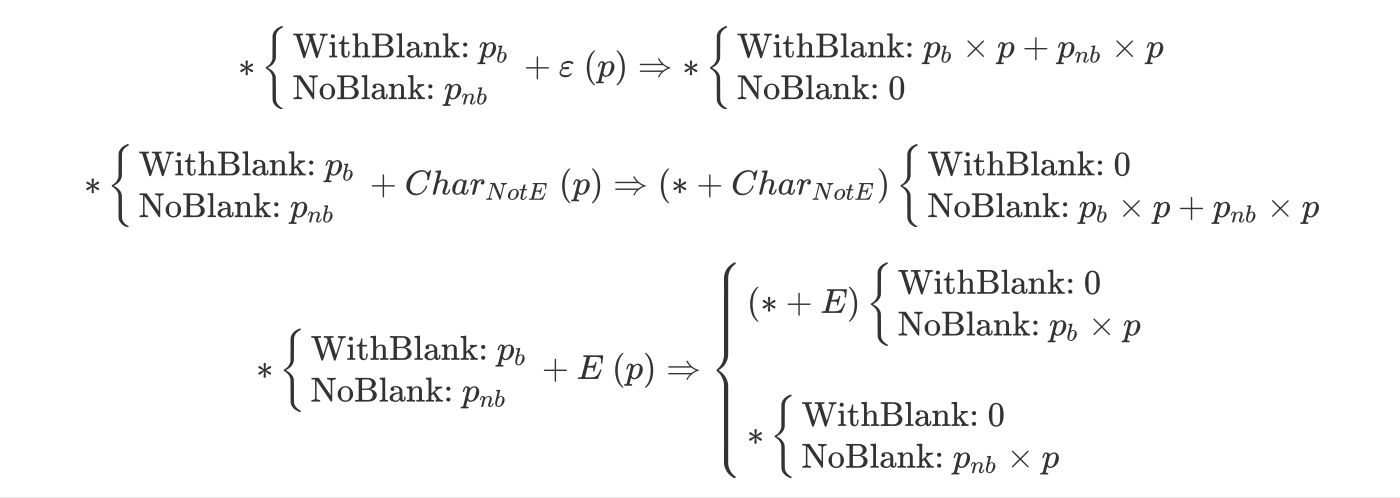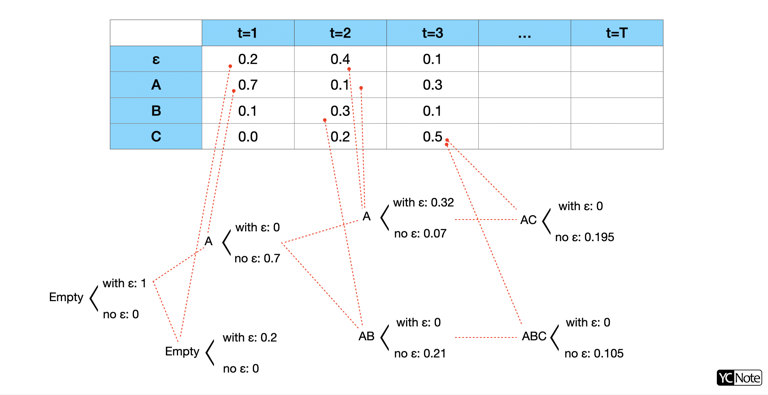1 2 3 4 5 6 7 8 9 10 11 12 13 14 15 16 17 18 19 20 21 22 23 24 25 26 27 28 29 30 31 32 33 34 35 36 37 38 39 40 41 42 43 44 45 46 47 48 49 50 51 52 53 54 55 56 def prefix_beam_decode(emission_log_prob, blank=0, **kwargs): beam_size = kwargs['beam_size'] emission_threshold = kwargs.get('emission_threshold', np.log(DEFAULT_EMISSION_THRESHOLD)) length, class_count = emission_log_prob.shape beams = [(tuple(), (0, NINF))] # (prefix, (blank_log_prob, non_blank_log_prob)) # initial of beams: (empty_str, (log(1.0), log(0.0))) for t in range(length): new_beams_dict = defaultdict(lambda: (NINF, NINF)) # log(0.0) = NINF for prefix, (lp_b, lp_nb) in beams: for c in range(class_count): log_prob = emission_log_prob[t, c] if log_prob < emission_threshold: continue end_t = prefix[-1] if prefix else None # if new_prefix == prefix new_lp_b, new_lp_nb = new_beams_dict[prefix] if c == blank: new_beams_dict[prefix] = ( logsumexp([new_lp_b, lp_b + log_prob, lp_nb + log_prob]), new_lp_nb ) continue if c == end_t: new_beams_dict[prefix] = ( new_lp_b, logsumexp([new_lp_nb, lp_nb + log_prob]) ) # if new_prefix == prefix + (c,) new_prefix = prefix + (c,) new_lp_b, new_lp_nb = new_beams_dict[new_prefix] if c != end_t: new_beams_dict[new_prefix] = ( new_lp_b, logsumexp([new_lp_nb, lp_b + log_prob, lp_nb + log_prob]) ) else: new_beams_dict[new_prefix] = ( new_lp_b, logsumexp([new_lp_nb, lp_b + log_prob]) ) # sorted by log(blank_prob + non_blank_prob) beams = sorted(new_beams_dict.items(), key=lambda x: logsumexp(x), reverse=True) beams = beams[:beam_size] labels = list(beams) return labels 

## 結語

 1 2 3 4 git clone https://github.com/GitYCC/crnn-pytorch.git cd crnn-pytorch/ pip install -r requirements.txt python src/predict.py demo/*.jpg